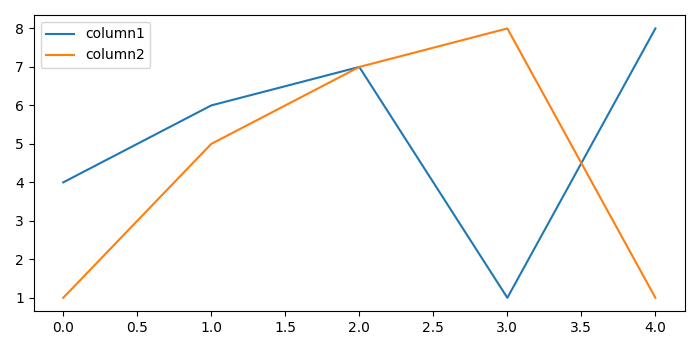# How to get rid of grid lines when plotting with Seaborn + Pandas with secondary_y?

To get rid of grid lines when plotting with Pandas with secondary_y, we can take the following steps −

• Create a data frame using DataFrame wth keys column1 and column2.

• Use data frame data to plot the data frame. To get rid of gridlines, use grid=False.

• To display the figure, use show() method.

## Example

import pandas as pd
from matplotlib import pyplot as plt
plt.rcParams["figure.figsize"] = [7.00, 3.50]
plt.rcParams["figure.autolayout"] = True
data = pd.DataFrame({"column1": [4, 6, 7, 1, 8], "column2": [1, 5, 7, 8, 1]})
data.plot(secondary_y=, grid=False)
plt.show()

## Output